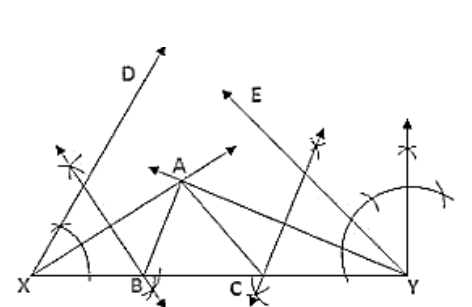# Using rulers and compasses only, construct a △ABC from the following data:

Question:

Using rulers and compasses only, construct a △ABC from the following data: AB + BC + CA = 12 cm, ∠B = 45° and ∠C = 60°

Solution:Steps of Construction:

1. Draw a line segment XY of 12 cm.

2. Draw ∠DXY = 45° and ∠EYX = 60°.

3. Draw the angle bisectors of ∠DXY and ∠EYX which intersect each other at A.

4. Draw the perpendicular bisector of AX and AY which intersect XY at B and C respectively.

5. Join AB and AC.

Hence △ABC is the required triangle.